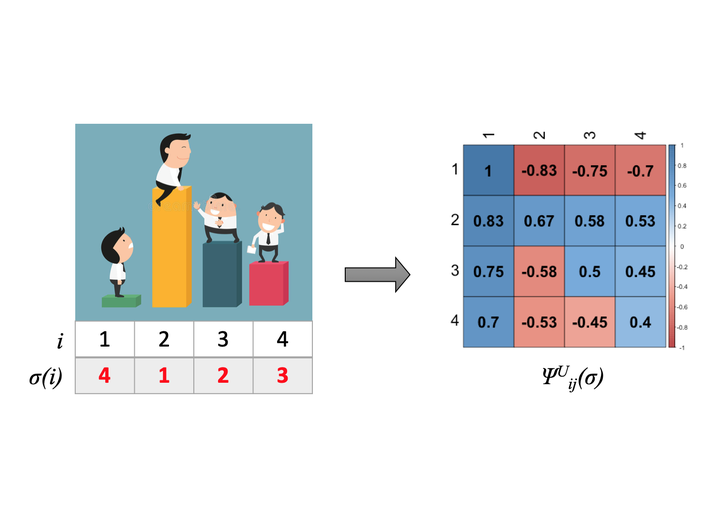# The Weighted Kendall and High-order Kernels for PermutationsWeighted Kendall embedding of a permutation.

### Abstract

We propose new positive definite kernels for permutations. First we introduce a weighted version of the Kendall kernel, which allows to weight unequally the contributions of different item pairs in the permutations depending on their ranks. Like the Kendall kernel, we show that the weighted version is invariant to relabeling of items and can be computed efficiently in $O(n \ln(n))$ operations, where $n$ is the number of items in the permutation. Second, we propose a supervised approach to learn the weights by jointly optimizing them with the function estimated by a kernel machine. Third, while the Kendall kernel considers pairwise comparison between items, we extend it by considering higher-order comparisons among tuples of items and show that the supervised approach of learning the weights can be systematically generalized to higher-order permutation kernels.

Type
Publication
In Proceedings of the 35th International Conference on Machine Learning (ICML)##### Yunlong Jiao
###### Applied Machine Learning Research

My research interests include Deep Generative Models, Vision Language Models, Natural Language Processing, and Computational Biology.